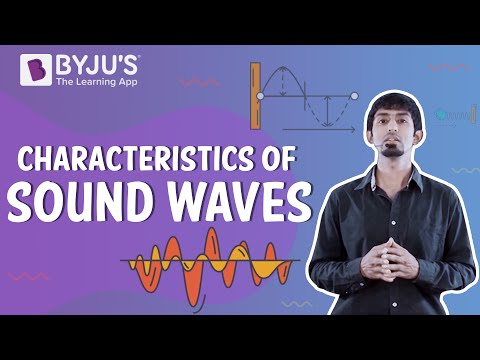# Unit of Wavelength

Wavelength is one of the important characteristics of a waveform apart from amplitude, velocity and frequency.

## What Is Wavelength?

Wavelength can be defined as the distance between two successive crests or troughs of a wave. It is the space generated in a periodic wave—the distance over which the wave’s shape is repeated. It is usually measured or determined by taking the distance between corresponding points of the same phase or different spatial wave patterns.

## Symbol And Units of Wavelength

Wavelength is commonly expressed by the symbol λ, which is actually a Greek letter known as lambda. Now that we have covered what is a wavelength, let’s get into the units.

Following is the table with different units of wavelength:

 SI unit of wavelength metre (m) CGS unit of wavelength centimetre (cm) Dimensional formula M0L1T0

## SI Unit of Wavelength

The SI unit of wavelength is metre usually denoted as m. While measuring wavelength the multiples or fractions of a metre is also used. Notably, exponential powers of 10 are used when wavelengths are of a large property. When there are shorter wavelengths they are expressed as negative exponential.

Meanwhile, wavelength also shares a great relationship with frequency. For instance, when the frequency increases, the wavelength decreases. You can find out more about the two properties by clicking the link given above.

Related articles:

Stay tuned with BYJU’S for more such interesting articles. Also, register to “BYJU’S – The Learning App” for loads of interactive, engaging Physics-related videos and unlimited academic assistance.

## The video about the characteristics of sound waves## Frequently Asked Questions – FAQs

Q1

### What is frequency?

Frequency is defined as the number of oscillations of a wave per unit time being measured in hertz(Hz). The frequency is directly proportional to the pitch. Humans can hear sounds with frequencies ranging between 20 – 20000 Hz.
Q2

### What is wavelength?

Wavelength is defined as the property of a wave in which the distance between the identical points between the two successive waves are calculated. It is denoted by the Greek letter lambda (λ). Therefore, the distance between either one crest or trough of one wave and the next wave is known as wavelength.
Q3

### Define visible spectrum.

The visible spectrum is nothing but the observable region of the electromagnetic wave which is visible to human eyes. In the electromagnetic spectrum, the visible spectrum ranges from the infrared region to the UV region.
Q4

### What are the properties of the light waves emitted by the laser?

Following are the properties of the light waves emitted by the laser:
• They have the same phase
• They have the same amplitude
• They have the same frequency
Q5

### What are electromagnetic waves?

Electromagnetic waves are also known as EM waves. Electromagnetic radiation is composed of electromagnetic waves that are produced when an electric field comes in contact with the magnetic field.
Test your Knowledge on unit of wavelength